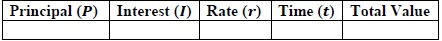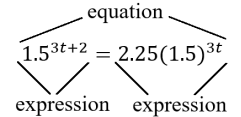# MA.912.FL.3.2Export Print
Solve real-world problems involving simple, compound and continuously compounded interest.

### Examples

Example: Find the amount of money on deposit at the end of 5 years if you started with \$500 and it was compounded quarterly at 6% interest per year.

Example: Joe won \$25,000 on a lottery scratch-off ticket. How many years will it take at 6% interest compounded yearly for his money to double?

### Clarifications

Clarification 1: Within the Algebra 1 course, interest is limited to simple and compound.
General Information
Subject Area: Mathematics (B.E.S.T.)
Strand: Financial Literacy
Status: State Board Approved

## Benchmark Instructional Guide

### Terms from the K-12 Glossary

• Simple Interest

### Vertical Alignment

Previous Benchmarks

Next Benchmarks

### Purpose and Instructional Strategies

In grade 7, students solved problems involving simple interest. In Algebra I, students solve problems involving simple and compound interest, using arithmetic operations and graphing. In later courses, students will solve compound interest problems to determine lengths of time, including those that require the use of logarithms, and solve continuously compounded interest problems.
• In this benchmark, students will be introduced to the concepts of simple and compound interest and will solve real-world problems that feature them.
• Instruction compares the differences between simple and compound interest.
• The simple interest formula ($I$ = $p$$r$$t$) calculates only the interest earned over time. Each year’s interest is calculated from the initial principal, not the total value of the investment of that point in time.
• The simple interest amount formula ($A$ = $P$(1 + $r$$t$)) calculates the total value of an investment over time.
• The compound interest formula ($A$ = $P$(1 +  $\frac{\text{r}}{\text{n}}$)$n$$t$) also calculates the total value of an investment over time. Each month/year’s interest is calculated from the total value of the investment of that point in time.
• Compound interest problems presented for this benchmark may require students to generate equivalent expressions to identify and interpret certain parts of the context.
• For example, Jason deposits \$850 in an account that earns an annual interest rate of 4.8%. The interest is compounded monthly and Jason wants to determine the total amount interest he will earn in one year. With the given information, derive that the value of the account is equal to 850 (1 + $\frac{\text{0.048}}{\text{12}}$)12$t$. The expression can be 12 rewritten as 850[(1.004)12]$t$ leading to 850(1.049)$t$ to find that the total amount interest in a year would be approximately 4.9% of his initial investment.

### Common Misconceptions or Errors

• Some problems related to this standard may ask students for the interest earned over a period of time while others may ask for the account balance or total value of the investment over a period of time. Some students may miss this distinction and may always calculate total interest for simple interest problems and total value for compound interest problems. In these cases, point students back to the working of the problem and help them assess the reasonableness of their answers (MTR.6.1) in context.

### Strategies to Support Tiered Instruction

• Teacher provides a highlighter to identify if a question is asking for the interest or the total amount.
• Instruction provides a graphic organizer to identify the important information in a problem.
• For example, given a simple interest problem, students could complete the following table.• Instruction includes the opportunity to distinguish between an expression and an equation. These should be captured in a math journal.
• For example, when generating equivalent expressions, place an equal sign in between the expressions and label each expression and the equation.• Felipe signs up for a new airline credit card that has a 24% annual interest rate. If he doesn’t pay his monthly statements, interest on his balance would compound daily. If Felipe never pays his statements for a full year, what would be the actual percentage rate he would pay the credit card company?

• Gwen deposits \$800 in a savings account that pays simple annual interest. After 18 months, she earns \$64.80. What is the interest rate for her account?

• You deposit \$500 in a savings account that pays 2.2% simple annual interest. Find your account balance after 15 months.

### Instructional Items

Instruction Item 1
• Beatrice deposits \$525 in an account that pays 4.3% simple annual interest. If she keeps the money in the account for 12 years, how much interest will she earn?

*The strategies, tasks and items included in the B1G-M are examples and should not be considered comprehensive.

## Related Courses

This benchmark is part of these courses.
1200310: Algebra 1 (Specifically in versions: 2014 - 2015, 2015 - 2022, 2022 and beyond (current))
1200320: Algebra 1 Honors (Specifically in versions: 2014 - 2015, 2015 - 2022, 2022 and beyond (current))
1200330: Algebra 2 (Specifically in versions: 2014 - 2015, 2015 - 2022, 2022 and beyond (current))
1200340: Algebra 2 Honors (Specifically in versions: 2014 - 2015, 2015 - 2022, 2022 and beyond (current))
1200370: Algebra 1-A (Specifically in versions: 2014 - 2015, 2015 - 2022, 2022 and beyond (current))
1200380: Algebra 1-B (Specifically in versions: 2014 - 2015, 2015 - 2022, 2022 and beyond (current))
1200400: Foundational Skills in Mathematics 9-12 (Specifically in versions: 2014 - 2015, 2015 - 2022, 2022 and beyond (current))
7912070: Access Mathematics for Liberal Arts (Specifically in versions: 2014 - 2015, 2015 - 2018, 2018 - 2019, 2019 - 2022, 2022 - 2023, 2023 and beyond (current))
7912080: Access Algebra 1A (Specifically in versions: 2014 - 2015, 2015 - 2018, 2018 - 2019, 2019 - 2022, 2022 and beyond (current))
7912090: Access Algebra 1B (Specifically in versions: 2014 - 2015, 2015 - 2018, 2018 - 2019, 2019 - 2022, 2022 and beyond (current))
1200315: Algebra 1 for Credit Recovery (Specifically in versions: 2014 - 2015, 2015 - 2022, 2022 and beyond (current))
1200375: Algebra 1-A for Credit Recovery (Specifically in versions: 2014 - 2015, 2015 - 2022, 2022 and beyond (current))
1200385: Algebra 1-B for Credit Recovery (Specifically in versions: 2014 - 2015, 2015 - 2022, 2022 and beyond (current))
7912075: Access Algebra 1 (Specifically in versions: 2014 - 2015, 2015 - 2018, 2018 - 2019, 2019 - 2022, 2022 and beyond (current))
7912095: Access Algebra 2 (Specifically in versions: 2016 - 2018, 2018 - 2019, 2019 - 2022, 2022 and beyond (current))
1207350: Mathematics for College Liberal Arts (Specifically in versions: 2022 and beyond (current))
1200388: Mathematics for Data and Financial Literacy Honors (Specifically in versions: 2022 and beyond (current))
1200384: Mathematics for Data and Financial Literacy (Specifically in versions: 2022 and beyond (current))
7912120: Access Mathematics for Data and Financial Literacy (Specifically in versions: 2022 - 2023, 2023 and beyond (current))
2102300: Economics and Personal Finance (Specifically in versions: 2023 and beyond (current))
2102305: Economics and Personal Finance Honors (Specifically in versions: 2023 and beyond (current))
2102371: Personal Finance and Money Management (Specifically in versions: 2023 and beyond (current))
2102373: Personal Finance and Money Management Honors (Specifically in versions: 2023 and beyond (current))

## Related Access Points

Alternate version of this benchmark for students with significant cognitive disabilities.
MA.912.FL.3.AP.2: Solve real-world problems involving simple and compound interest.

## Related Resources

Vetted resources educators can use to teach the concepts and skills in this benchmark.

## Lesson Plan

How much is your time worth?:

This lesson is designed to help students solve real-world problems involving compound and continuously compounded interest. Students will also be required to translate word problems into function models, evaluate functions for inputs in their domains, and interpret outputs in context.

Type: Lesson Plan

## Student Resources

Vetted resources students can use to learn the concepts and skills in this benchmark.

## Parent Resources

Vetted resources caregivers can use to help students learn the concepts and skills in this benchmark.